# Intermediate Maths solutions for Ellipse

Inter Maths IIB solutions for Ellipse exercise 4(a) and 4(b) are given.

These solutions are very easy to understand. First you study the textbook lesson very well.

Then you observe the example problems and solutions. Try them.

Next try exercise problems. Observe the given solutions and try them in your own method.

You can see solutions for Inter text book Maths IIB

1. Circle

3. Parabola

4. Ellipse

You can also see solutions for Inter text book Maths IIA

For examination purpose you can see

Complex numbers

De Moivre’ s Theorem

# Inter maths solutions for Ellipse

Exercise 4(a)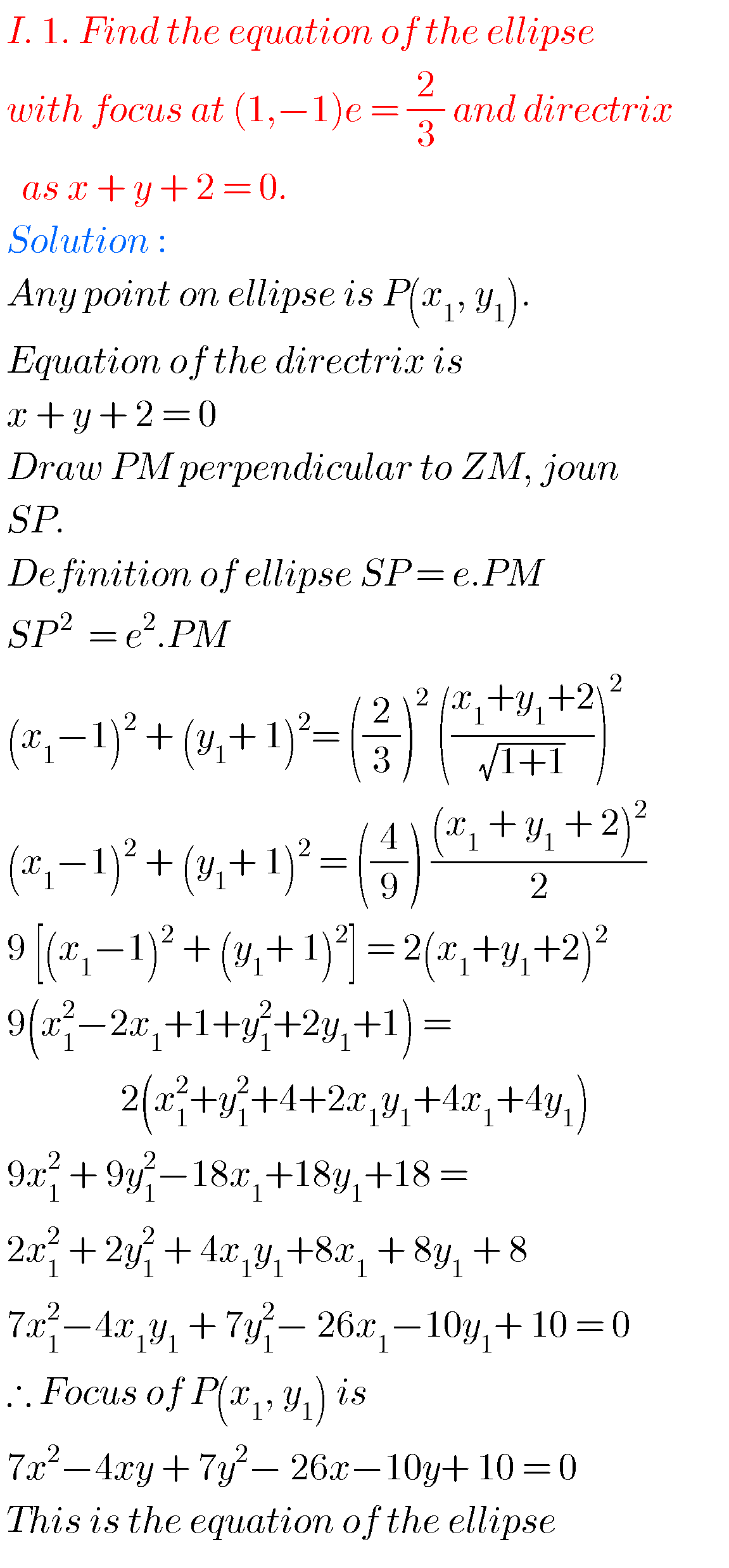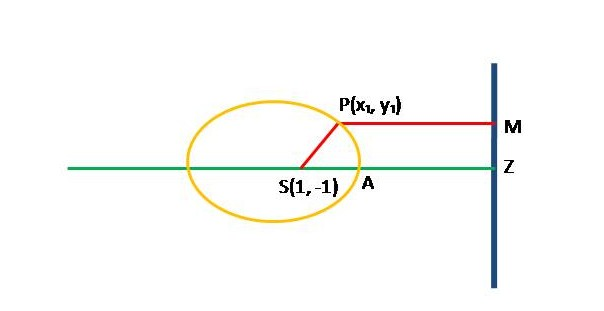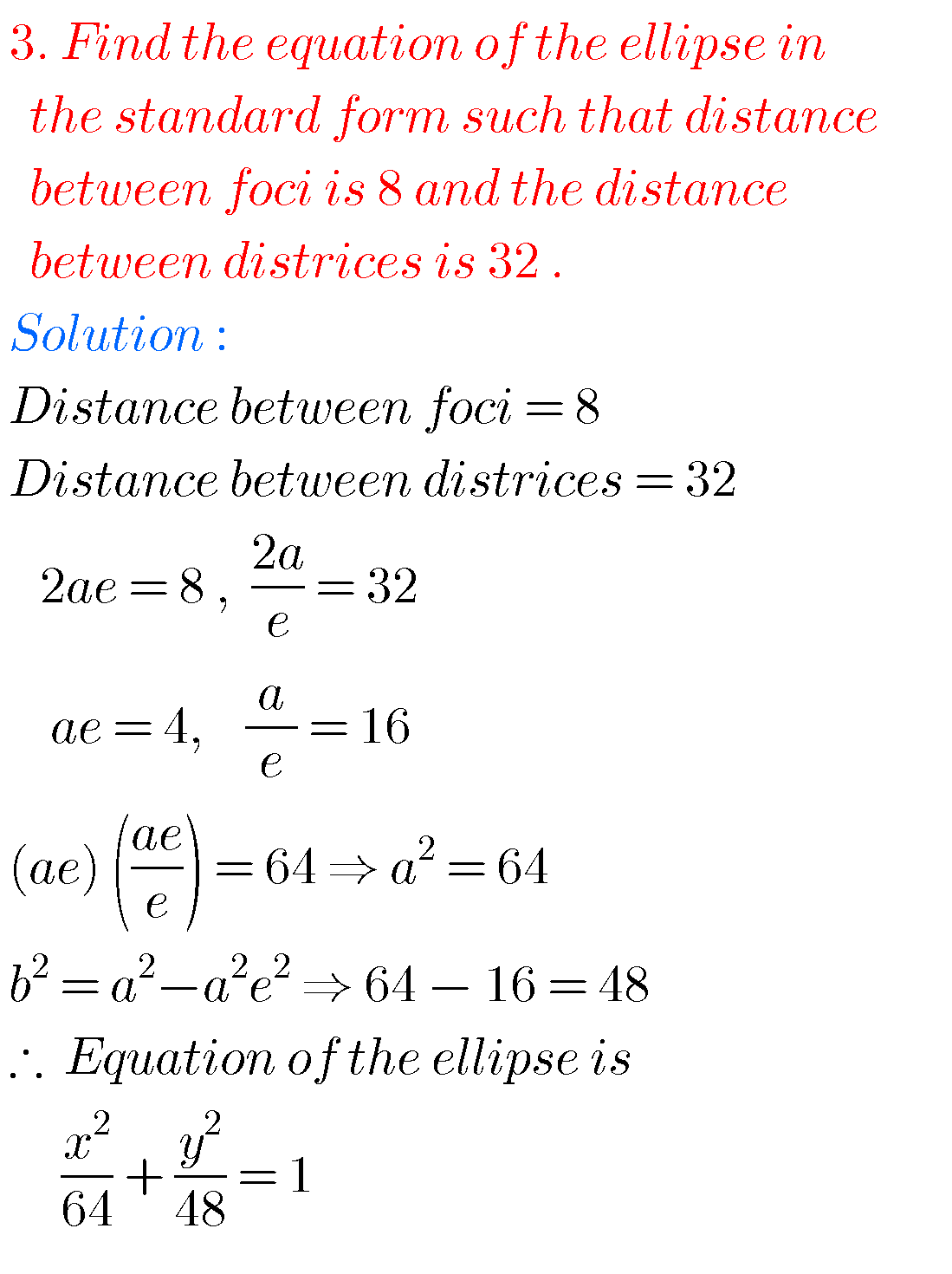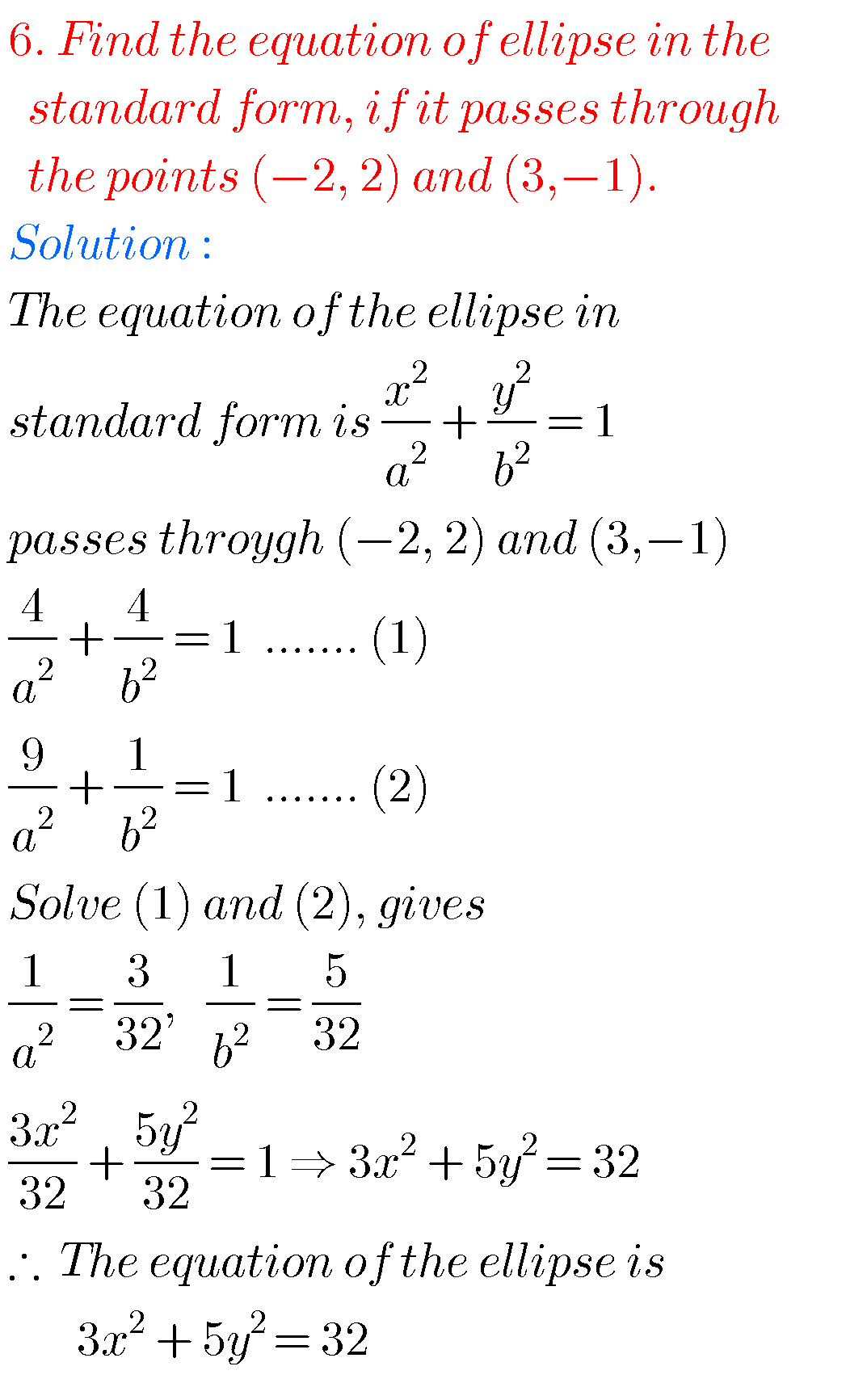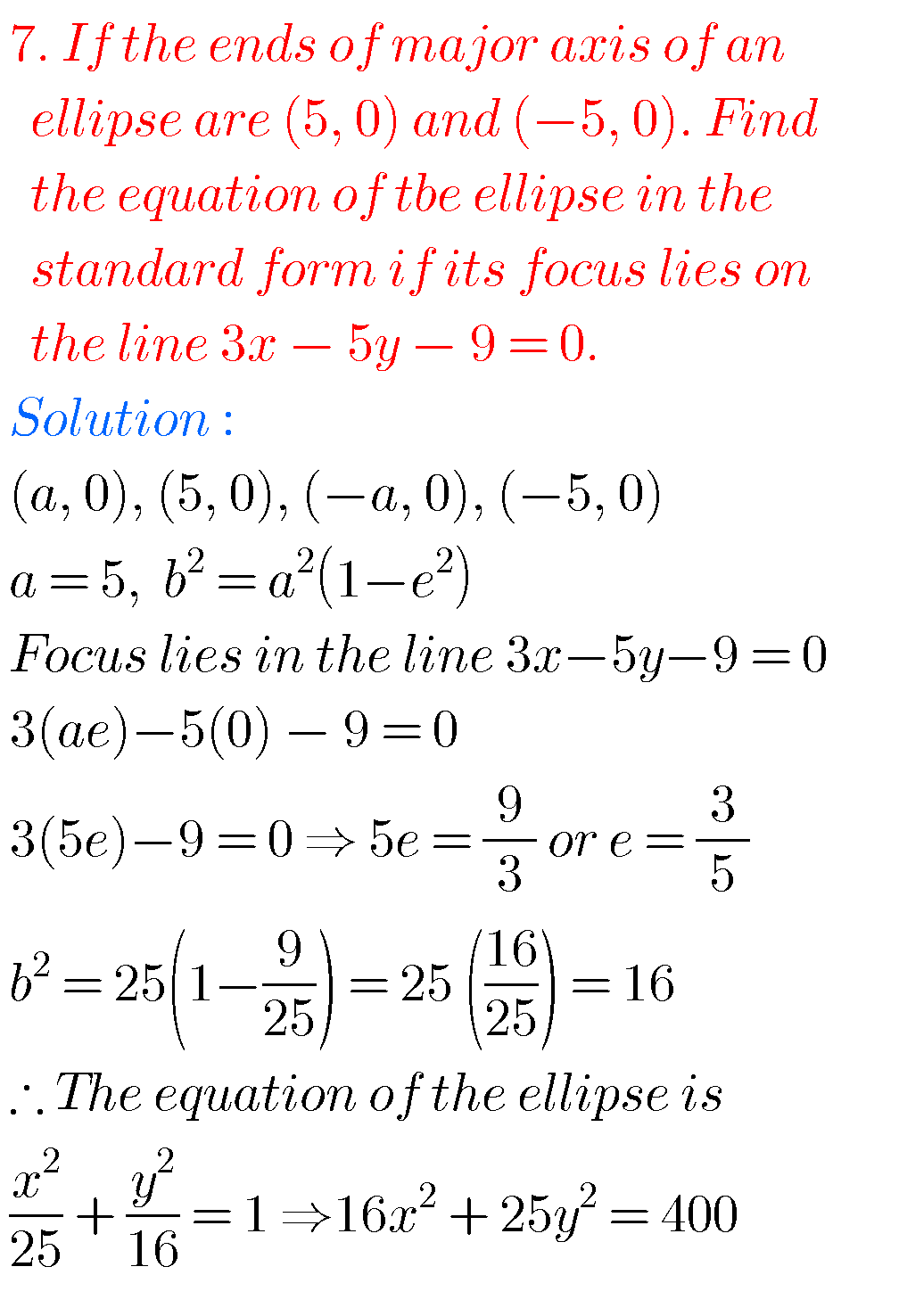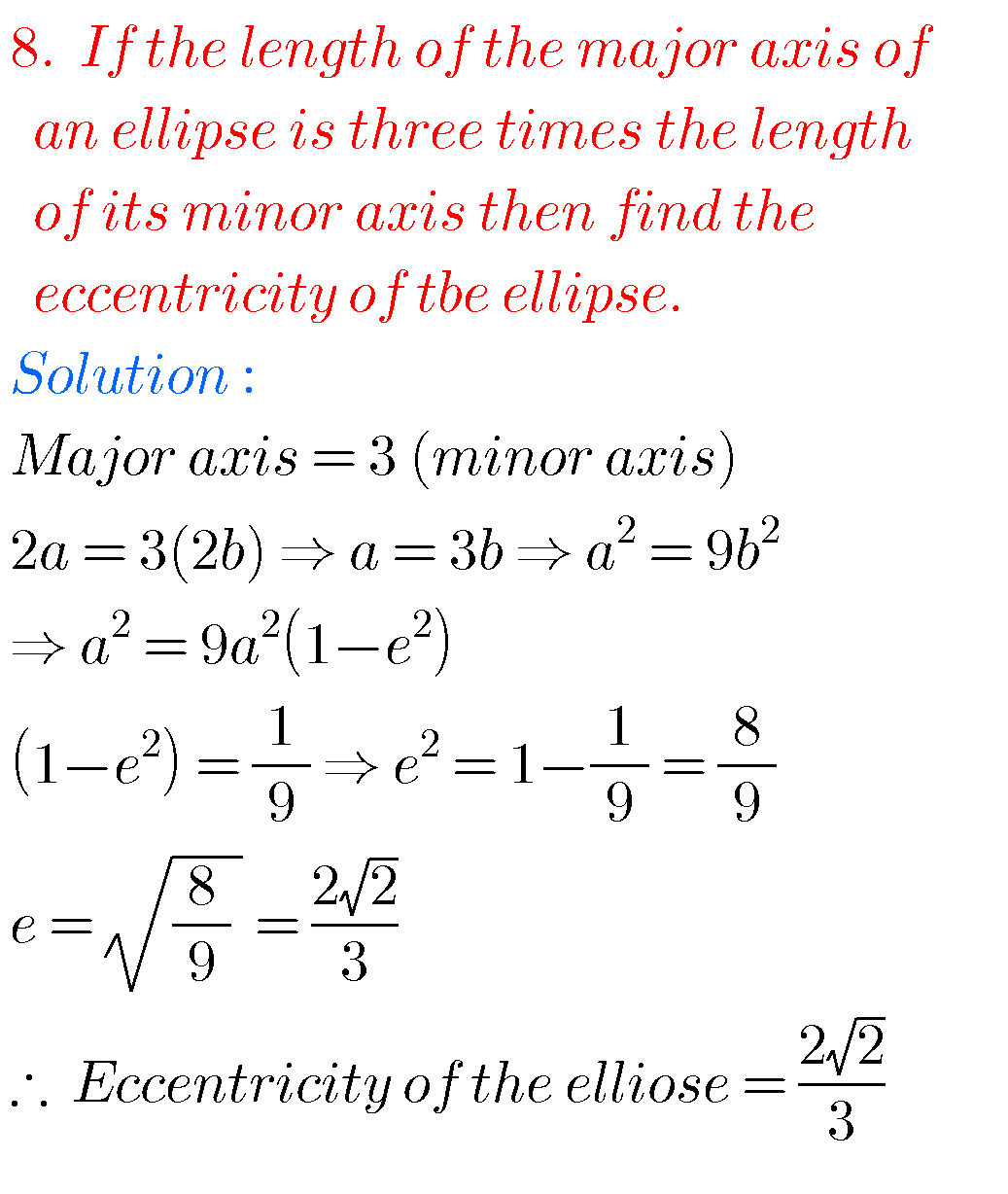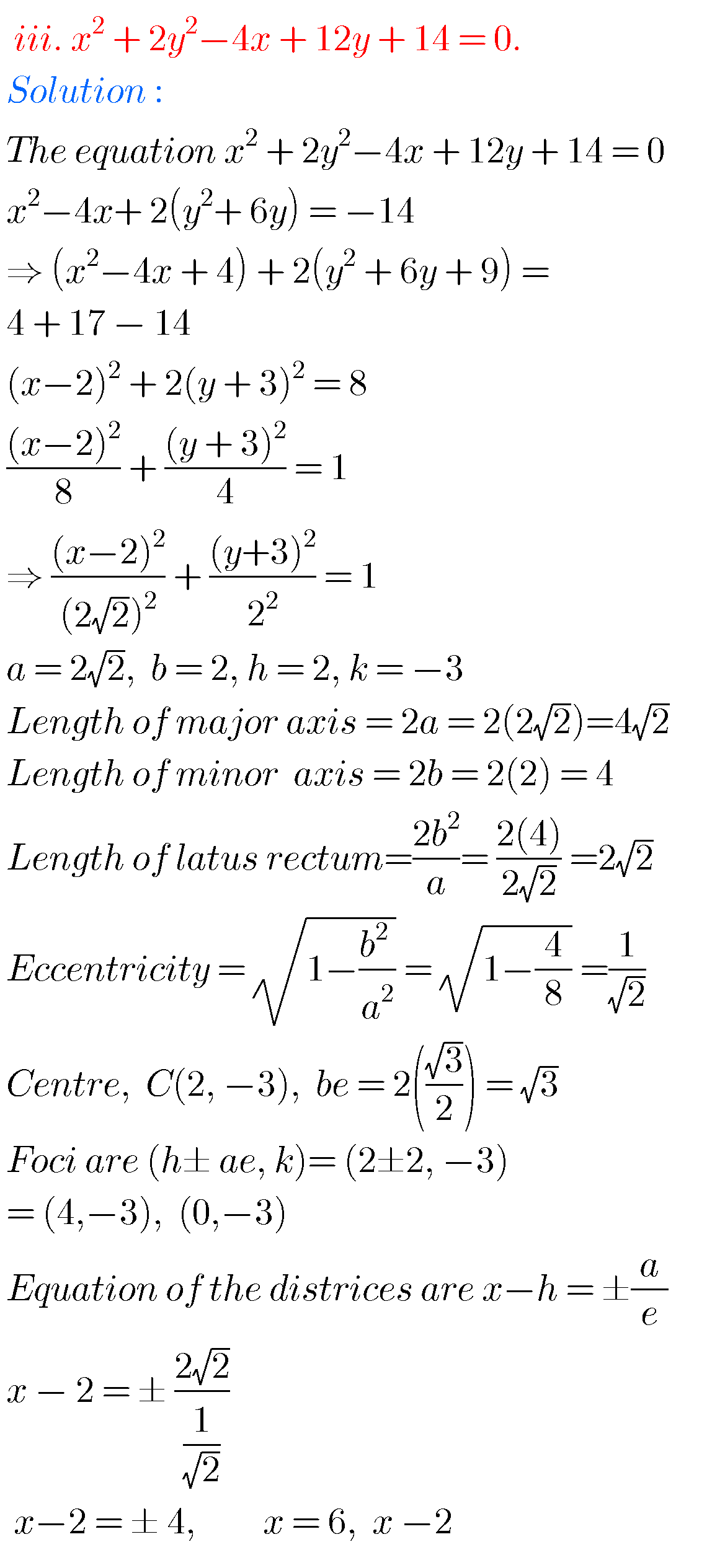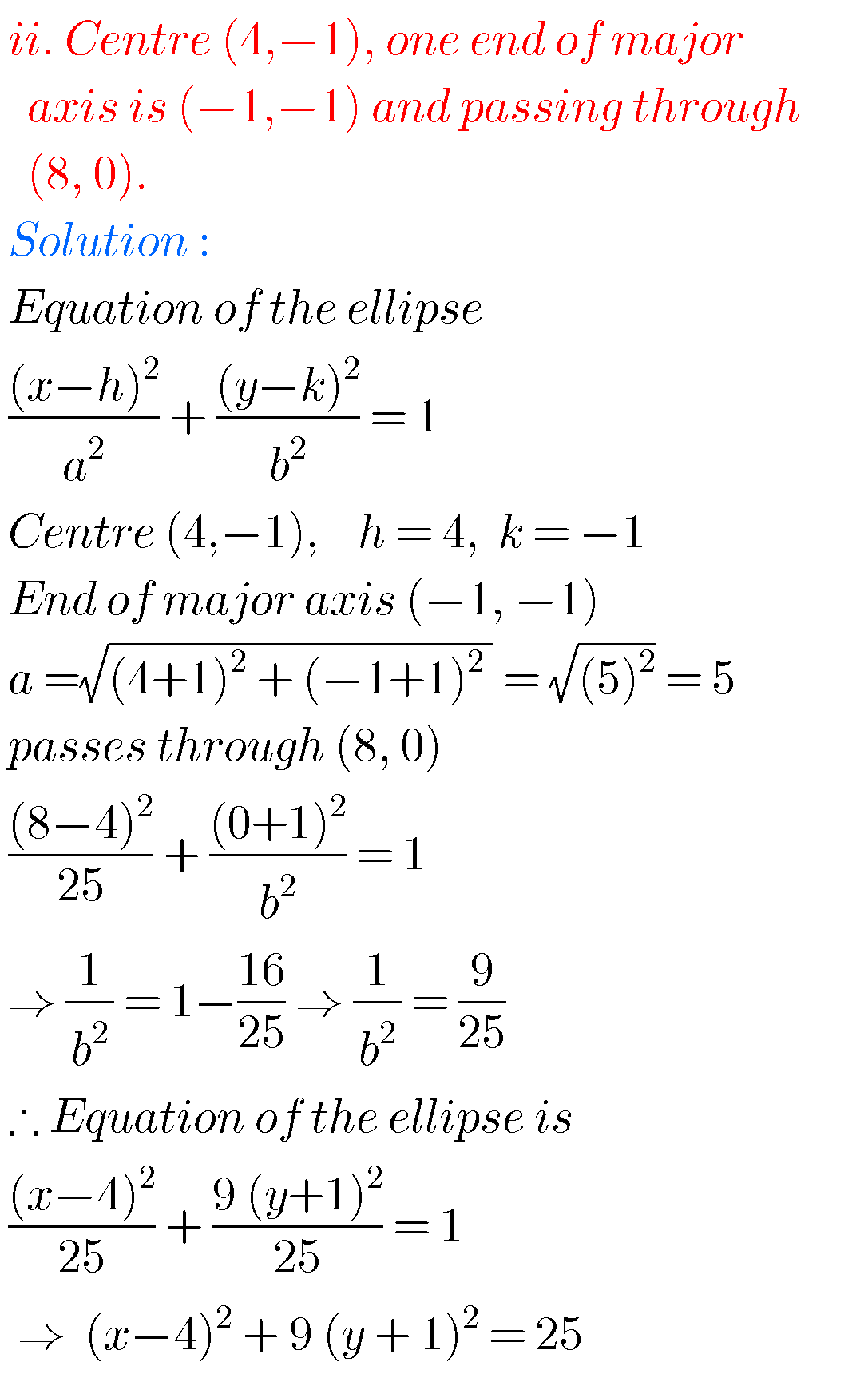## Ellipse solutions Inter Maths IIB

Exercise 4(b)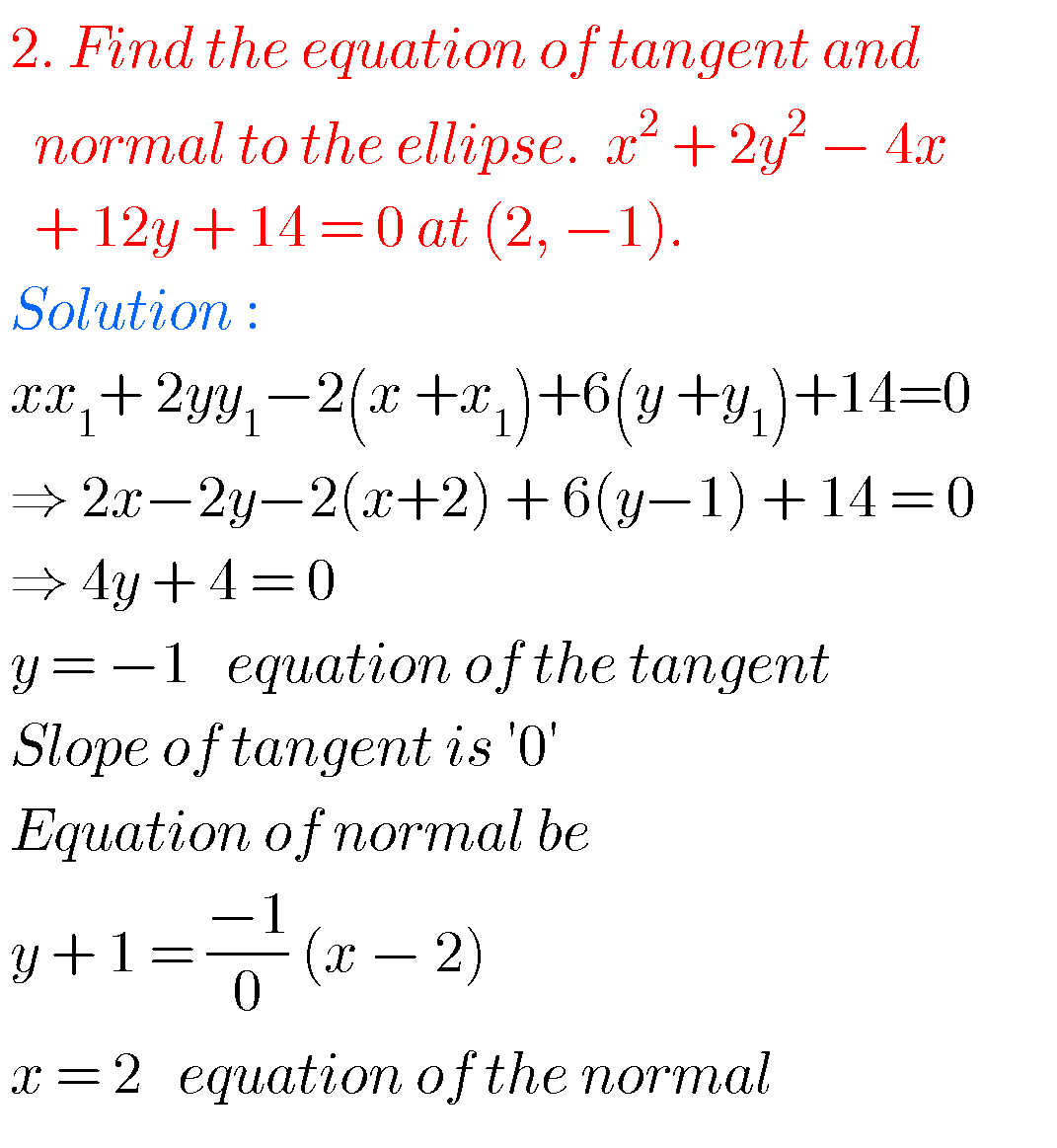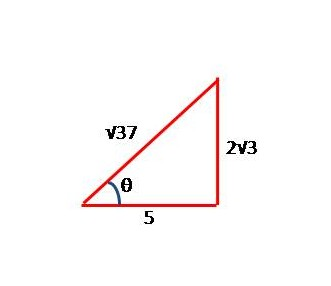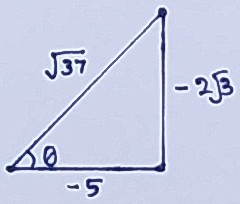### Maths 2b solutions for Ellipse Inter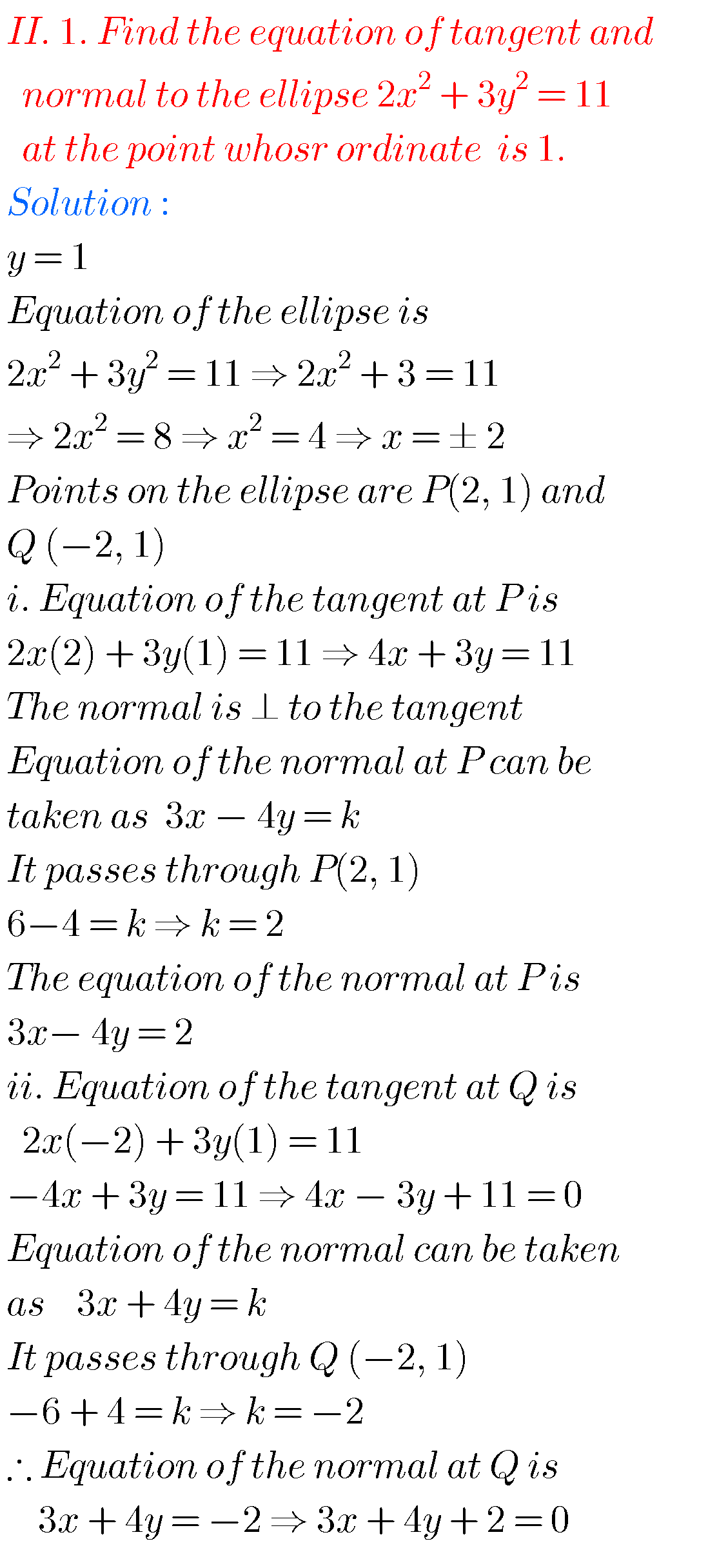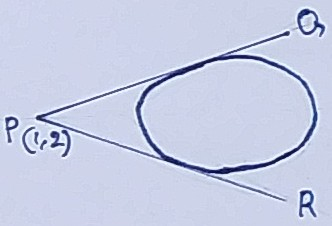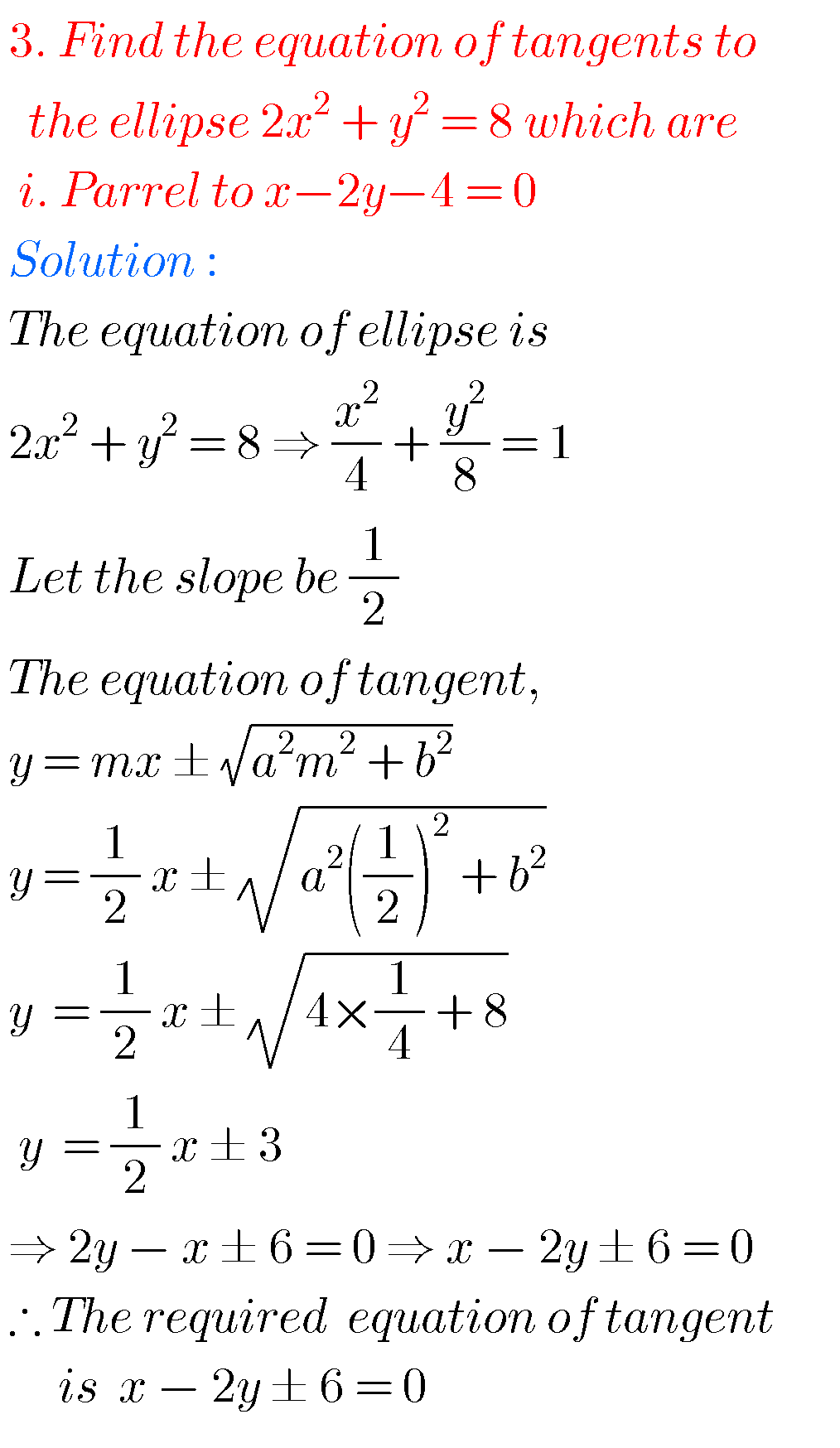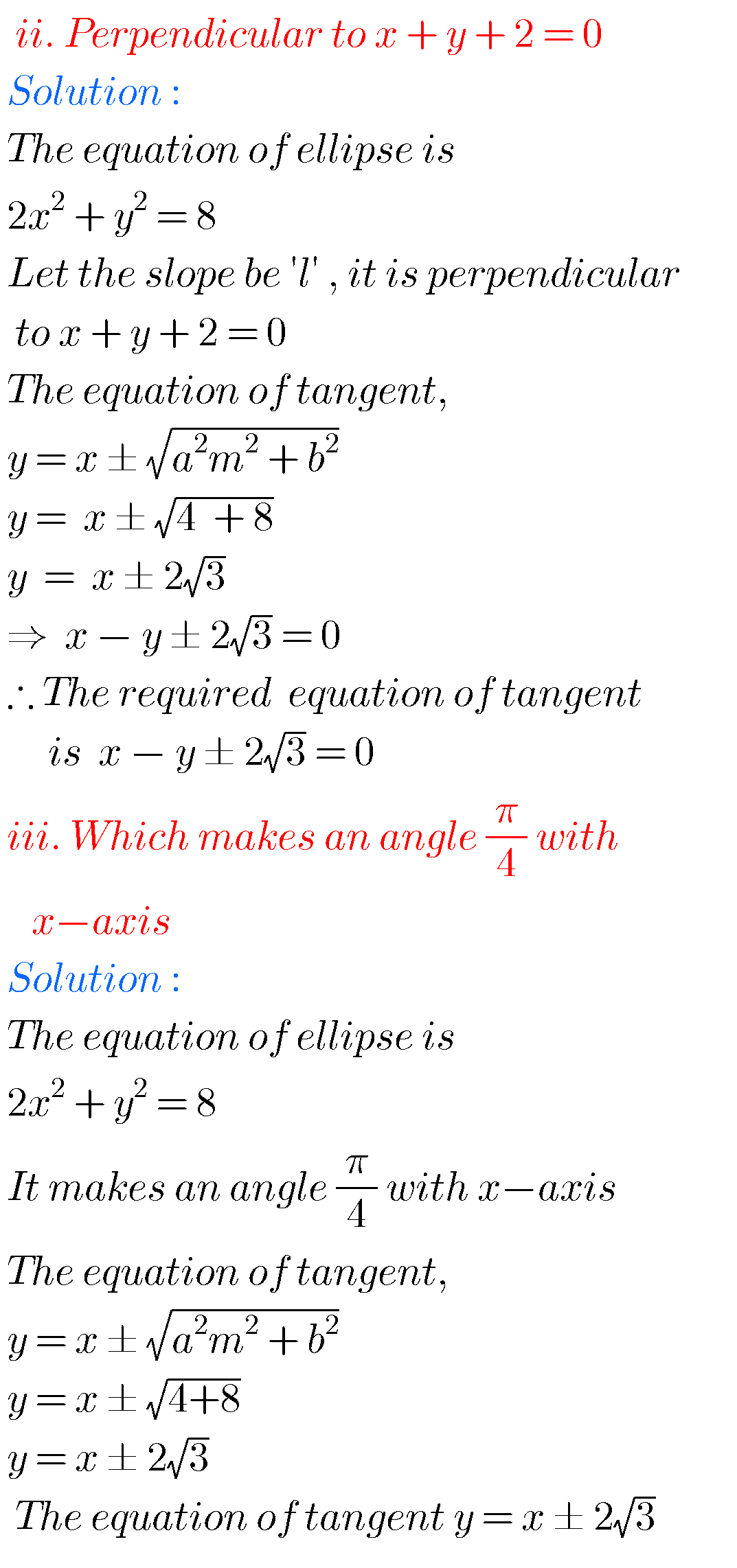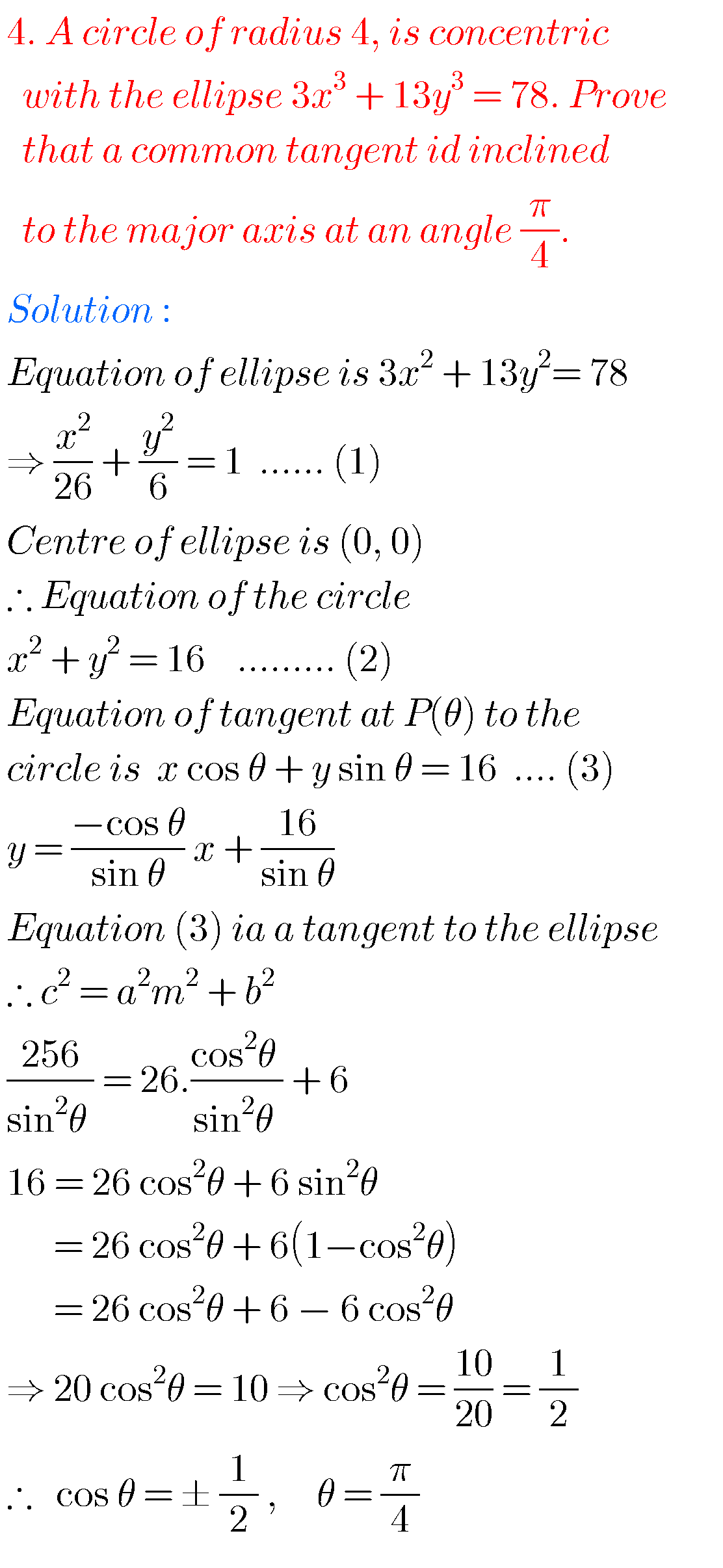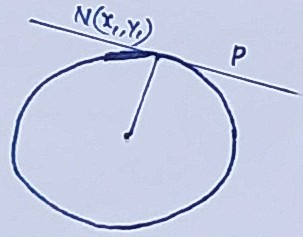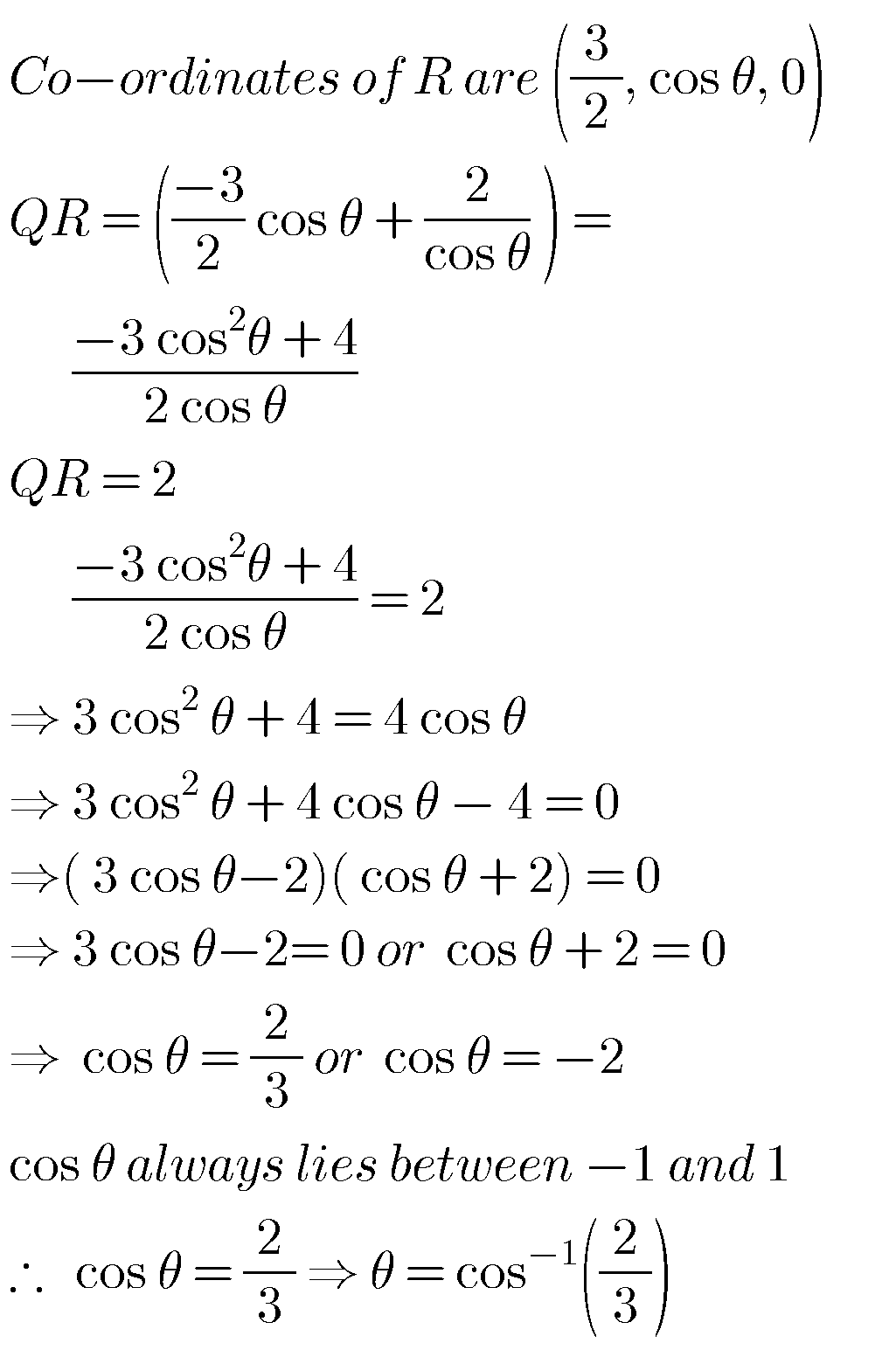Note : Observe the solutions and try them in your own method.

You can see solutions for Inter maths 1A for examination purpose

3. Matrices

You can also see solutions for Inter Maths 1B

1. Locus

You can see solutions for

Ncert solutions for class 7 integers

You can see the solutions for text book Maths 1A

Functions

Exercise 1(a)

Exercise 1(b)

Exercise 1(c)

Mathematical Induction

Exercise 2(a)

Matrices

Exercise 3(a)

Exercise 3(b)

Exercise 3(c)

Exercise 3(d)

Exercise 3(e)

Exercise 3(f)

Exercise 3(g)

Trigonometric Ratios up to Transformations

Exercise 6(a)

Exercise 6(b)

Exercise 6(c)

Exercise 6(d)

Exercise 6(e)

Exercise 6(f)

Trigonometric Equations

Exercise 7(a)

Inverse Trigonometric Equations

Exercise 8(a)

Hyperbolic Functions

Exercise 9(a)

Properties of Triangles

Exercise 10(a)

Exercise 10(b)

1b solutions inter

Locus

Exercise 1(a)

Transformation of axes

Exercise 2(a)

Three Dimensional Coordinates

Exercise 5(a)
Exercise 5(b)
Direction Cosines and Direction Ratios

Exercise 6(a)
Exercise 6(b)
The Plane

Exercise 7(a)

Limits and Continuity

Exercise 8(a)

Exercise 8(b)